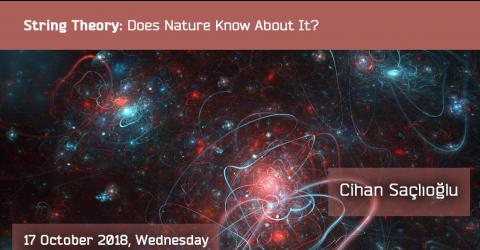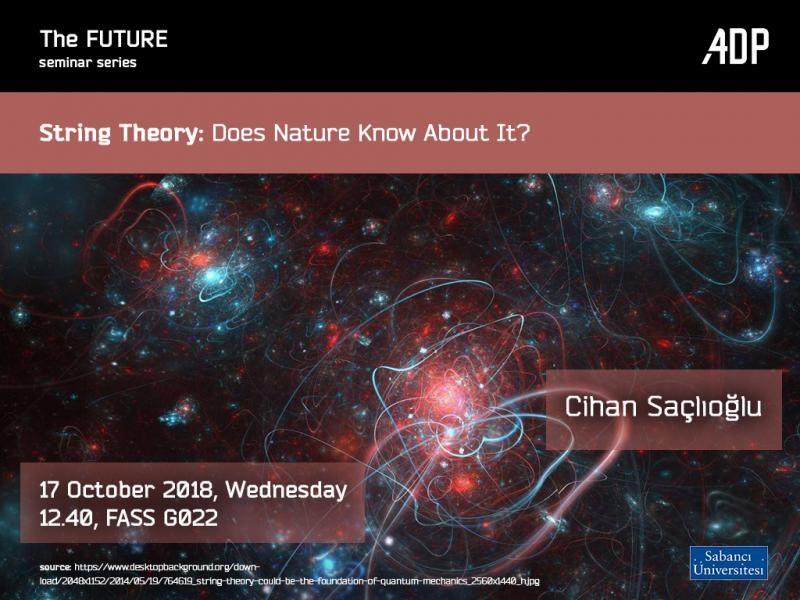FUTURE Seminar by Cihan SaçlıoğluDoes Nature Know about String Theory?

Cihan Saçlıoğlu

We can successfully describe three of the four fundamental interactions in Nature using the framework of quantum field theory. Gravity is the exception: although Einsteins 1915 Theory of General Theory of Relativity passes every experimental test, it is a classical field theory, much like Maxwell's theory of the electromagnetic field. Attempts to find its quantum version by the methods employed for the other three quantum field theories run into problems stemming from the interactions being assumed to happen at a mathematical point. In String Theory, point particles are replaced by strings that are a hundred billion billion times smaller than the atomic nucleus. Gravitons can be identified with closed strings of spin 2 and mass zero (photons have spin 1 and zero mass), and these couple to other matter as expected from Einstein's theory. In that sense, it can be said that "String theory predicts general relativity". On the other hand, space is required to have 25 or 9 or 10 dimensions. This can be handled in principle by assuming all but 3 space dimensions close upon themselves, but other problems remain. While the theory has been phenomenally successful as a mathematical tool, there is clearly much work to do in understanding its relevance to physics.